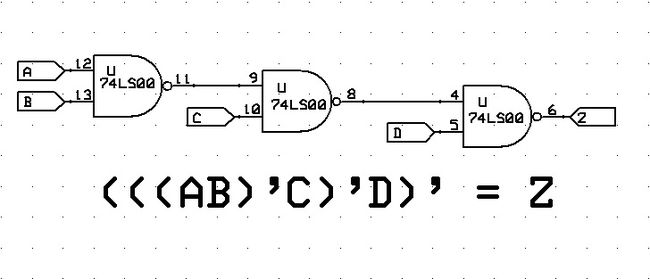# NAND Blocks$NAND$ and $NOR$ gates are said to be the building block gates of all digital logic circuits because all other logic gates can be implemented using only the combinations of multiple $NAND$ or $NOR$ gates.

What is the minimum number of $NAND$ gates needed to implement $XOR$ logic?